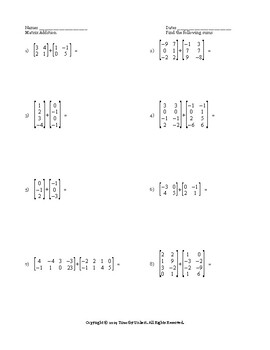Matrix Elements and OperationsSubject
Resource Type
Common Core State Standards
Product Rating
File Type

PDF (Acrobat) Document File

Be sure that you have an application to open this file type before downloading and/or purchasing.

80 KB|9 pages
Share
Also included in:
1. In this worksheet bundle of matrices students will identify elements of matrices, add matrices, subtract matrices, multiply matrices by a constant, multiply two matrices together, solve one and two-step matrix equations, find the determinants of 2 x 2 and 3 x 3 matrices, determine whether a matrices
\$5.49
\$4.94
Save \$0.55
Product Description

In this packet of worksheets students will tackle 42 challenging problems having them identify elements of a matrix, add matrices, subtract matrices, multiply a constant by a matrix, multiply two matrices together, and solve one and two step equations involving matrices.

42 problems, 9 pages, answer keys included. This can be used for in-class worksheets, a homework assignment, or even as assessments.

For more great PreCalculus material please check out my PreCalculus oriented website https://precalcgenius.com!

Total Pages
9 pages
Included
Teaching Duration
N/A
Report this Resource to TpT
Reported resources will be reviewed by our team. Report this resource to let us know if this resource violates TpT’s content guidelines.